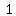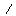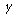# U+215F FRACTION NUMERATOR ONE

U+215F was added to Unicode in version 1.1 (1993). It belongs to the blockNumber Forms in the Basic Multilingual Plane.

This character is a Other Number and is commonly used, that is, in no specific script. The codepoint has the Numeric value 1.

In mathematics, a multiplicative inverse or reciprocal for a number x, denoted by 1/x or x−1, is a number which when multiplied by x yields the multiplicative identity, 1. The multiplicative inverse of a fraction a/b is b/a. For the multiplicative inverse of a real number, divide 1 by the number. For example, the reciprocal of 5 is one fifth (1/5 or 0.2), and the reciprocal of 0.25 is 1 divided by 0.25, or 4. The reciprocal function, the function f(x) that maps x to 1/x, is one of the simplest examples of a function which is its own inverse (an involution).

The term reciprocal was in common use at least as far back as the third edition of Encyclopædia Britannica (1797) to describe two numbers whose product is 1; geometrical quantities in inverse proportion are described as reciprocall in a 1570 translation of Euclid's Elements.

In the phrase multiplicative inverse, the qualifier multiplicative is often omitted and then tacitly understood (in contrast to the additive inverse). Multiplicative inverses can be defined over many mathematical domains as well as numbers. In these cases it can happen that abba; then "inverse" typically implies that an element is both a left and right inverse.

The notation f −1 is sometimes also used for the inverse function of the function f, which is not in general equal to the multiplicative inverse. For example, the multiplicative inverse 1/(sin x) = (sin x)−1 is different from the inverse sin of x, denoted sin−1 x or arcsin x. Only for linear maps are they strongly related (see below). The terminology difference reciprocal versus inverse is not sufficient to make this distinction, since many authors prefer the opposite naming convention, probably for historical reasons (for example in French, the inverse function is preferably called application réciproque).

## Representations

System Representation
8543
UTF-8 E2 85 9F
UTF-16 21 5F
UTF-32 00 00 21 5F
URL-Quoted %E2%85%9F
HTML-Escape &#x215F;
Wrong windows-1252 Mojibake â

## Complete Record

Property Value
Age (age) 1.1
Unicode Name (na) FRACTION NUMERATOR ONE
Unicode 1 Name (na1)
Block (blk) Number_Forms
General Category (gc) Other Number
Script (sc) Common
Bidirectional Category (bc) Other Neutral
Combining Class (ccc) Not Reordered
Decomposition Type (dt) Fraction
Decomposition Mapping (dm)Lowercase (Lower)
Simple Lowercase Mapping (slc)Lowercase Mapping (lc)Uppercase (Upper)
Simple Uppercase Mapping (suc)Uppercase Mapping (uc)Simple Titlecase Mapping (stc)Titlecase Mapping (tc)Case Folding (cf)ASCII Hex Digit (AHex)
Alphabetic (Alpha)
Bidi Control (Bidi_C)
Bidi Mirrored (Bidi_M)
Bidi Paired Bracket (bpb)Bidi Paired Bracket Type (bpt) None
Cased (Cased)
Composition Exclusion (CE)
Case Ignorable (CI)
Full Composition Exclusion (Comp_Ex)
Changes When Casefolded (CWCF)
Changes When Casemapped (CWCM)
Changes When NFKC Casefolded (CWKCF)
Changes When Lowercased (CWL)
Changes When Titlecased (CWT)
Changes When Uppercased (CWU)
Dash (Dash)
Deprecated (Dep)
Default Ignorable Code Point (DI)
Diacritic (Dia)
East Asian Width (ea) Neutral
Extender (Ext)
FC NFKC Closure (FC_NFKC)Grapheme Cluster Break (GCB) Any
Grapheme Base (Gr_Base)
Grapheme Extend (Gr_Ext)
Hex Digit (Hex)
Hangul Syllable Type (hst) Not Applicable
Hyphen (Hyphen)
ID Continue (IDC)
Ideographic (Ideo)
ID Start (IDS)
IDS Binary Operator (IDSB)
IDS Trinary Operator and (IDST)
InMC (InMC)
Indic Positional Category (InPC) NA
Indic Syllabic Category (InSC) Other
ISO 10646 Comment (isc)
Joining Group (jg) No_Joining_Group
Join Control (Join_C)
Jamo Short Name (JSN)
Joining Type (jt) Non Joining
Line Break (lb) Alphabetic
Logical Order Exception (LOE)
Math (Math)
Noncharacter Code Point (NChar)
NFC Quick Check (NFC_QC) Yes
NFD Quick Check (NFD_QC) Yes
NFKC Casefold (NFKC_CF)NFKC Quick Check (NFKC_QC) No
NFKD Quick Check (NFKD_QC) No
Numeric Type (nt) Numeric
Numeric Value (nv) 1
Other Alphabetic (OAlpha)
Other Default Ignorable Code Point (ODI)
Other Grapheme Extend (OGr_Ext)
Other ID Continue (OIDC)
Other ID Start (OIDS)
Other Lowercase (OLower)
Other Math (OMath)
Other Uppercase (OUpper)
Pattern Syntax (Pat_Syn)
Pattern White Space (Pat_WS)
Quotation Mark (QMark)
Sentence Break (SB) Other
Simple Case Folding (scf)Script Extension (scx) Common
Soft Dotted (SD)
STerm (STerm)
Terminal Punctuation (Term)
Unified Ideograph (UIdeo)
Variation Selector (VS)
Word Break (WB) Other
White Space (WSpace)
XID Continue (XIDC)
XID Start (XIDS)
Expands On NFC (XO_NFC)
Expands On NFD (XO_NFD)
Expands On NFKC (XO_NFKC)
Expands On NFKD (XO_NFKD)